Regression Tutorial 2: Principal Components Analysis (PCA)

This tutorial is optional

• PCA is a very common “dimension reduction” tool
• It can take several correlated variables and extact a smaller number of uncorrelated, “latent” variables
• The math behind this is tricky, and the interpretation requires some practice
• Getting good at PCA let’s you explore the structure of complex datasets
• For the milk dataset, I found that Australian mammals are very distinct from all other species. This was alluded to in the original paper but did not factor into their analysis.
• We’ll briefly do 2 PCAs
• 1st on the reponse variables (y) from the milk dataset (% fat, % protein, etc) and
• 2nd on the predictor variables (body mass, duration of gestation etc)
• This is just a very very very brief intro to the R functions and key vocab. There are several good tutorials online with more info.

• pairs
• princomp
• biplot

Set working directory

setwd("C:/Users/lisanjie2/Desktop/TEACHING/1_STATS_CalU/1_STAT_CalU_2016_by_NLB/1_STAT_Duq_2017/HOMEWORK/Duq_Biostats_Homework_4_Milk_regression/homework4_RMD")

Load data cleaned in previous tutorial

PCA on predictor (x) variables

• The predictors result in a PCA that is a bit easier to interpret

Transformations

Because the variables are on very different scales it helps to transform them

dat\$gest.mo.l10 <- log10(dat\$gest.month.NUM)
dat\$repro.l10 <- log10(dat\$repro.output)
dat\$mass.litter.l10 <- log10(dat\$mass.litter)
dat\$lact.mo.l10 <- log10(dat\$lacatation.months)
dat\$mass.fem.l10 <- log10(dat\$mass.female)

Pairs plot

• Look for correlations among variables.
• Many of the predictors are highly correlated.
• Such collinearity among variables makes it difficult to build models w/multiple predictors
• Instead you can only use one predictor at a time
• These plots also indicate at curious cloud of points that typically occurs below the main point mass.
pairs(dat[,c("gest.mo.l10",
"repro.l10",
"mass.litter.l10",
"lact.mo.l10",
"mass.fem.l10")],
lower.panel = NULL)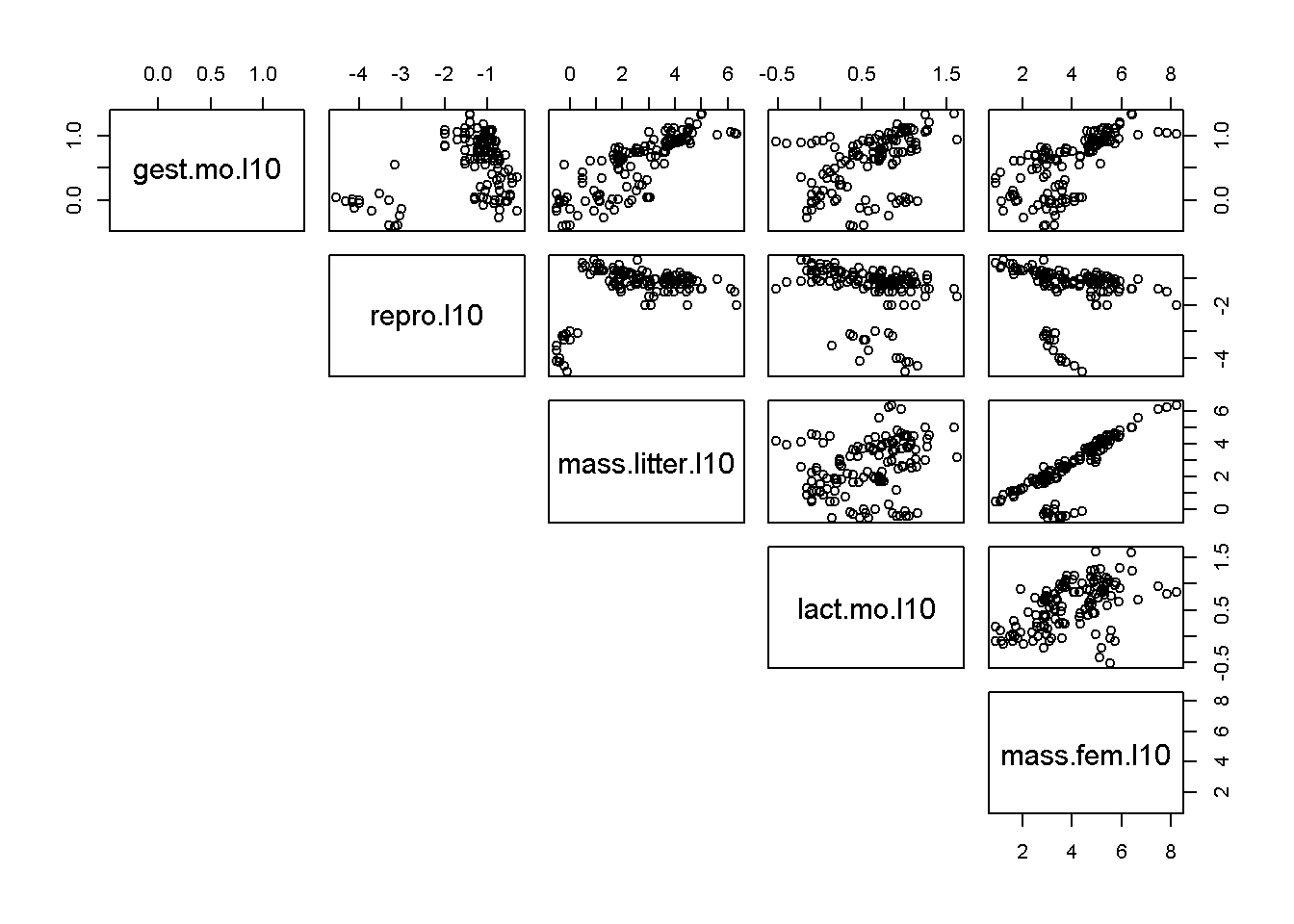PCA on predictory variables

Run the PCA with princomp()

#principal compoents
pca.x.vars <- princomp(~  gest.mo.l10+
repro.l10+
mass.litter.l10+
lact.mo.l10+
mass.fem.l10,
data = dat)

Biplot

A biplot takes some time to learn how to read, a skill that is beyond this tutorial. It is easy to see unique underlying structures pop out in biplots. The group of points away from the main band will be shown below to be Australian mammals (marsupials etc)

biplot(pca.x.vars)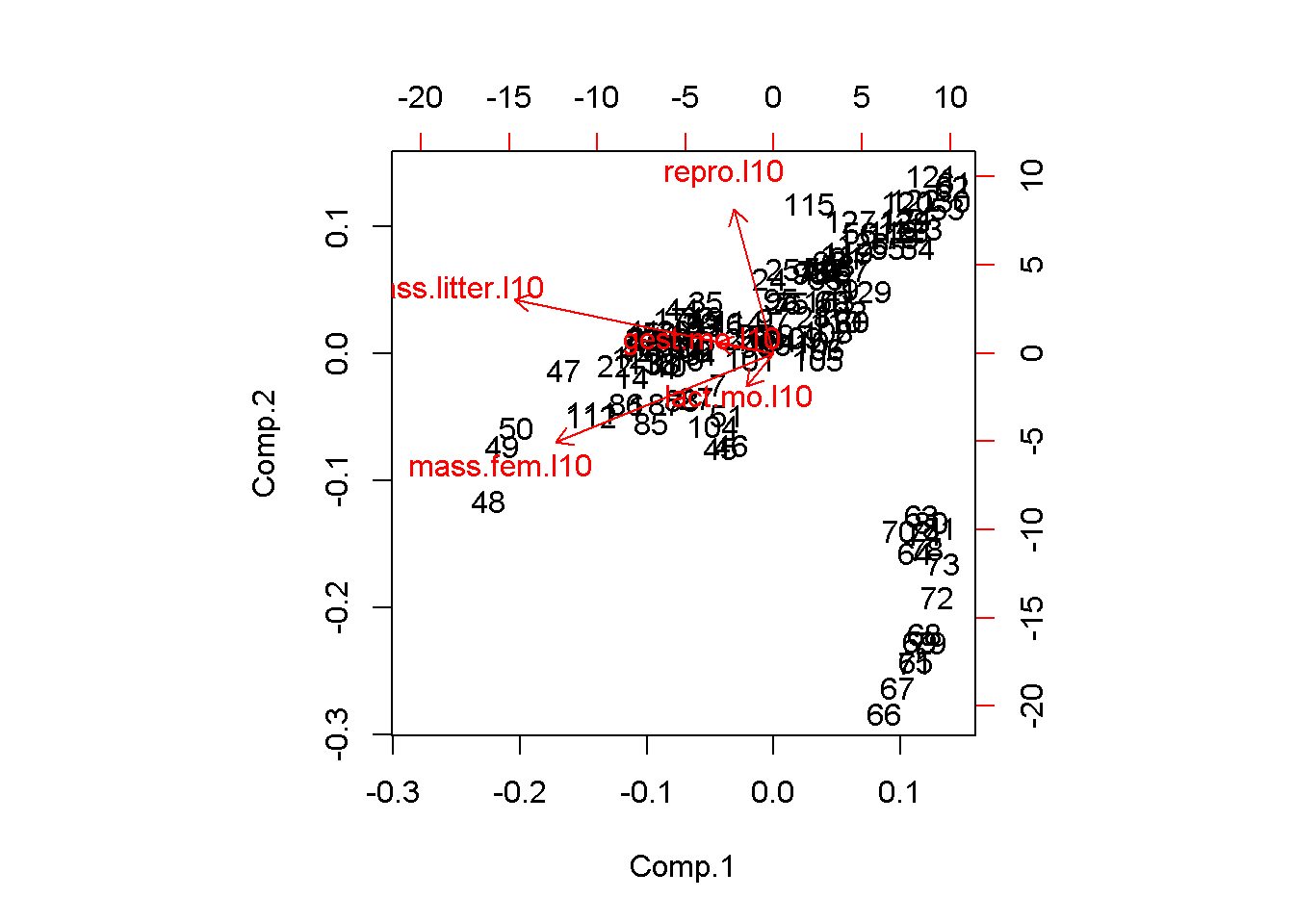Plot w/o point by setting first color to 0

biplot(pca.x.vars,col = c(0,2))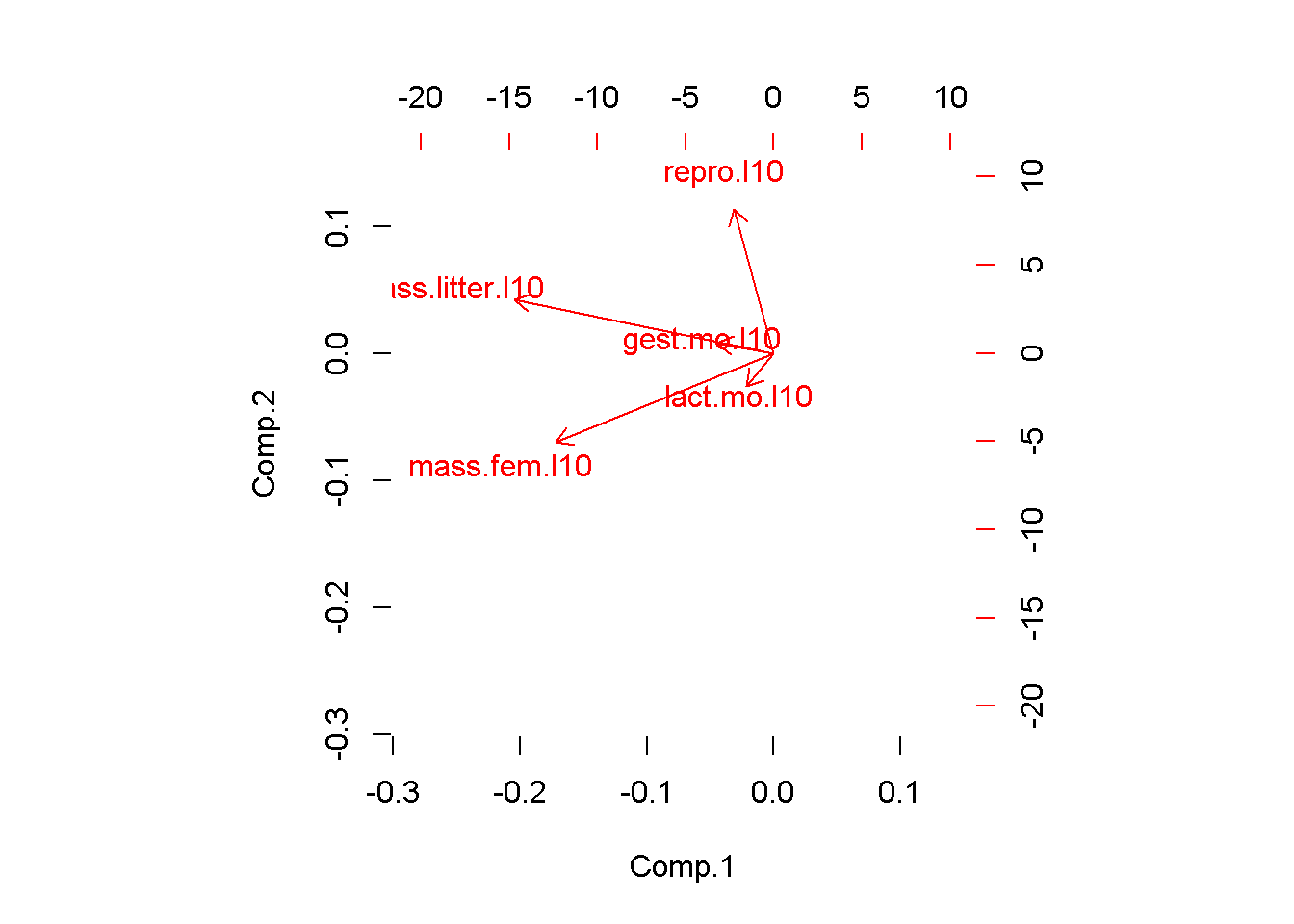The biplot tells us that a major axis of variation is female mass and lactation duration. These two variables are highly correlated. A 2nd major axis of variation is reproductive output, which is at a right angle to female mass/lactation. This implies that after accounting for female mass, there is additional, independent variation in the data due to reproduction. Litter size and gestation duration are at about a 45 degree angle between female maass reproduction. This implies another dimension of variation that is partially correlated with both of the other two.

PCA generates as many latent variables as there are original variables. Usually only the first few are useful. This codes displays the 2nd vs. the 3rd PCs

biplot(pca.x.vars, choices = c(2,3), col = c(0,2))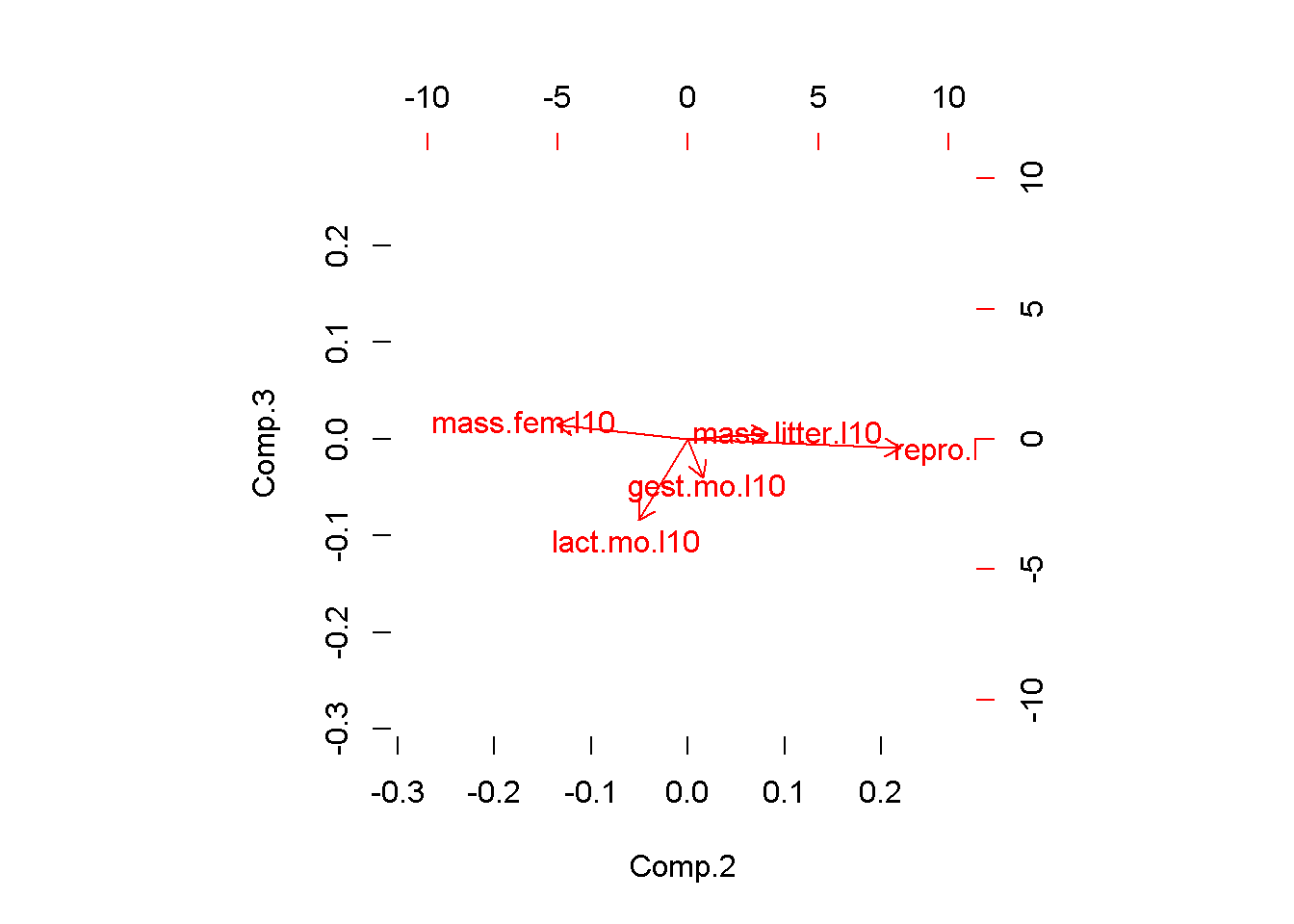What a biplot is plotting are “PCA” scores. We can plot these by hand by extracting them from the list embedded in the PCA output object. I’ll use qplot to plot

library(ggplot2)
qplot(y = pca.x.vars\$scores[ ,"Comp.2"] ,
x = pca.x.vars\$scores[ ,"Comp.1"] )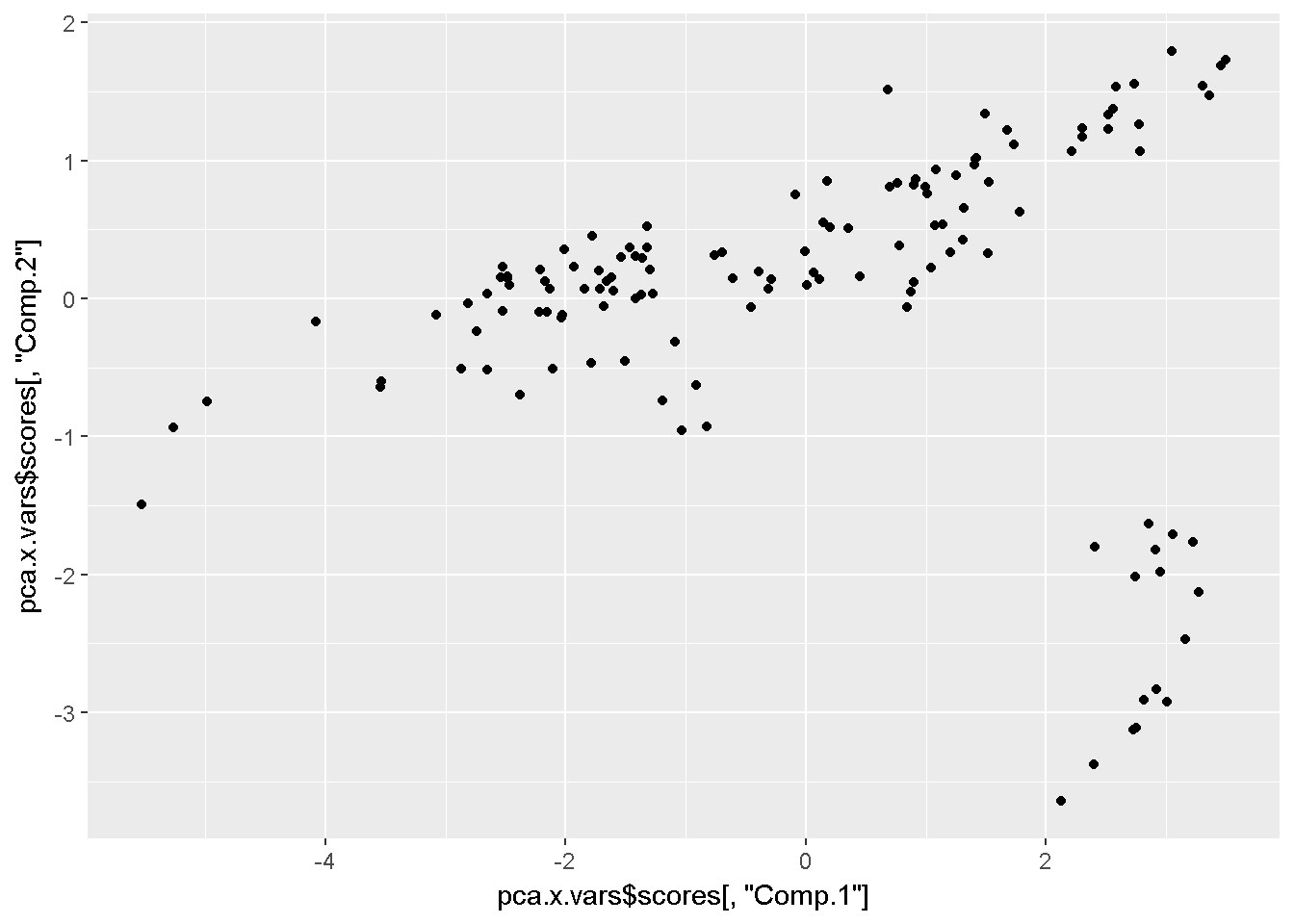And add color code them to identify groups. It looks like that outlying group are Monotremata. Diprotodontia (marsupials), and Didelphimorphia (opossum).

qplot(y = pca.x.vars\$scores[ ,"Comp.2"] ,
x = pca.x.vars\$scores[ ,"Comp.1"] ,
color = dat\$order)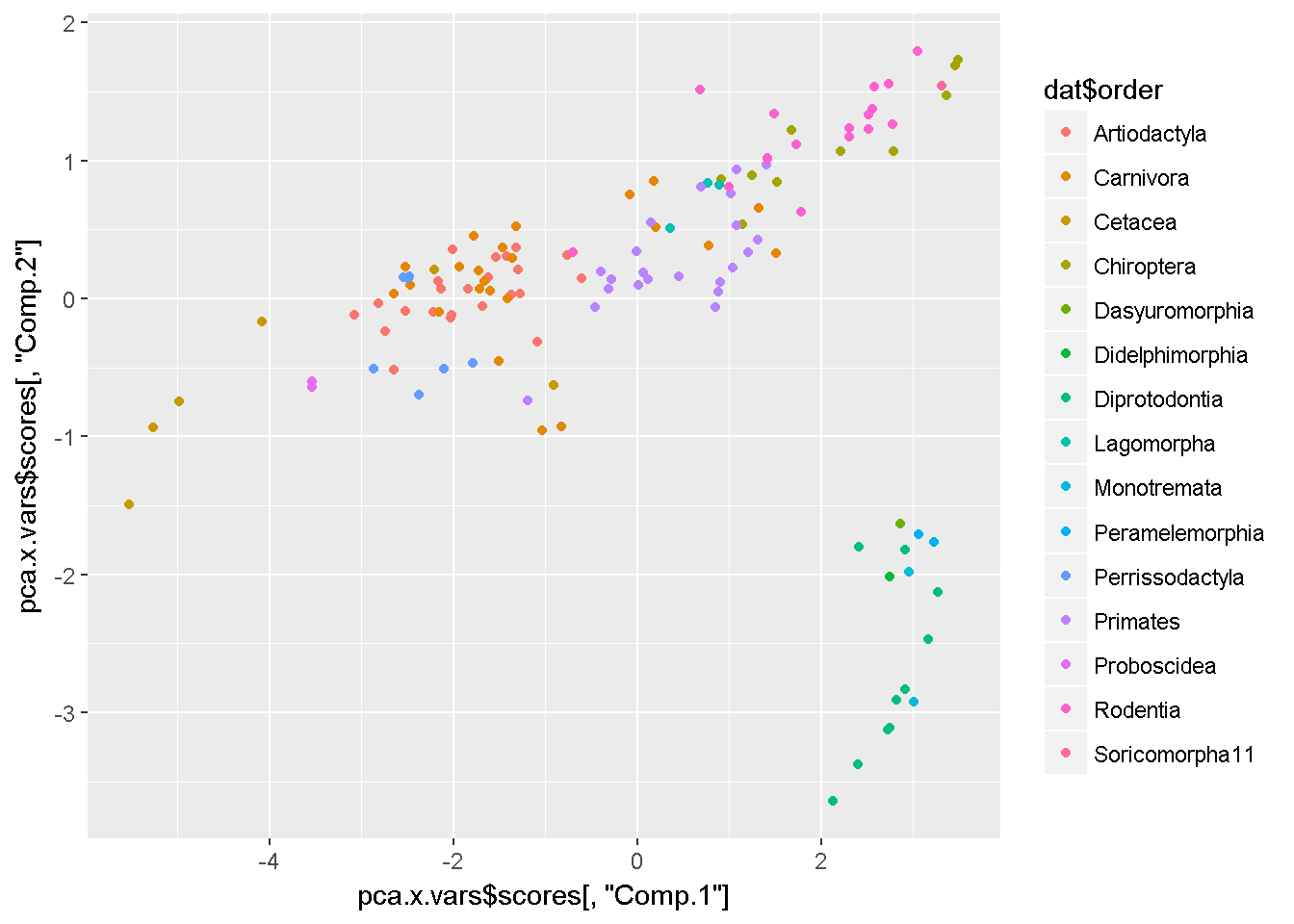I can add labels to confirm

labs <- gsub("ontia","",dat\$order)
labs <- gsub("ata","",dat\$order)

qplot(y = pca.x.vars\$scores[ ,"Comp.2"] ,
x = pca.x.vars\$scores[ ,"Comp.1"] ,
color = dat\$order) +
annotate(geom = "text",
y = pca.x.vars\$scores[ ,"Comp.2"] ,
x = pca.x.vars\$scores[ ,"Comp.1"] ,
label = labs)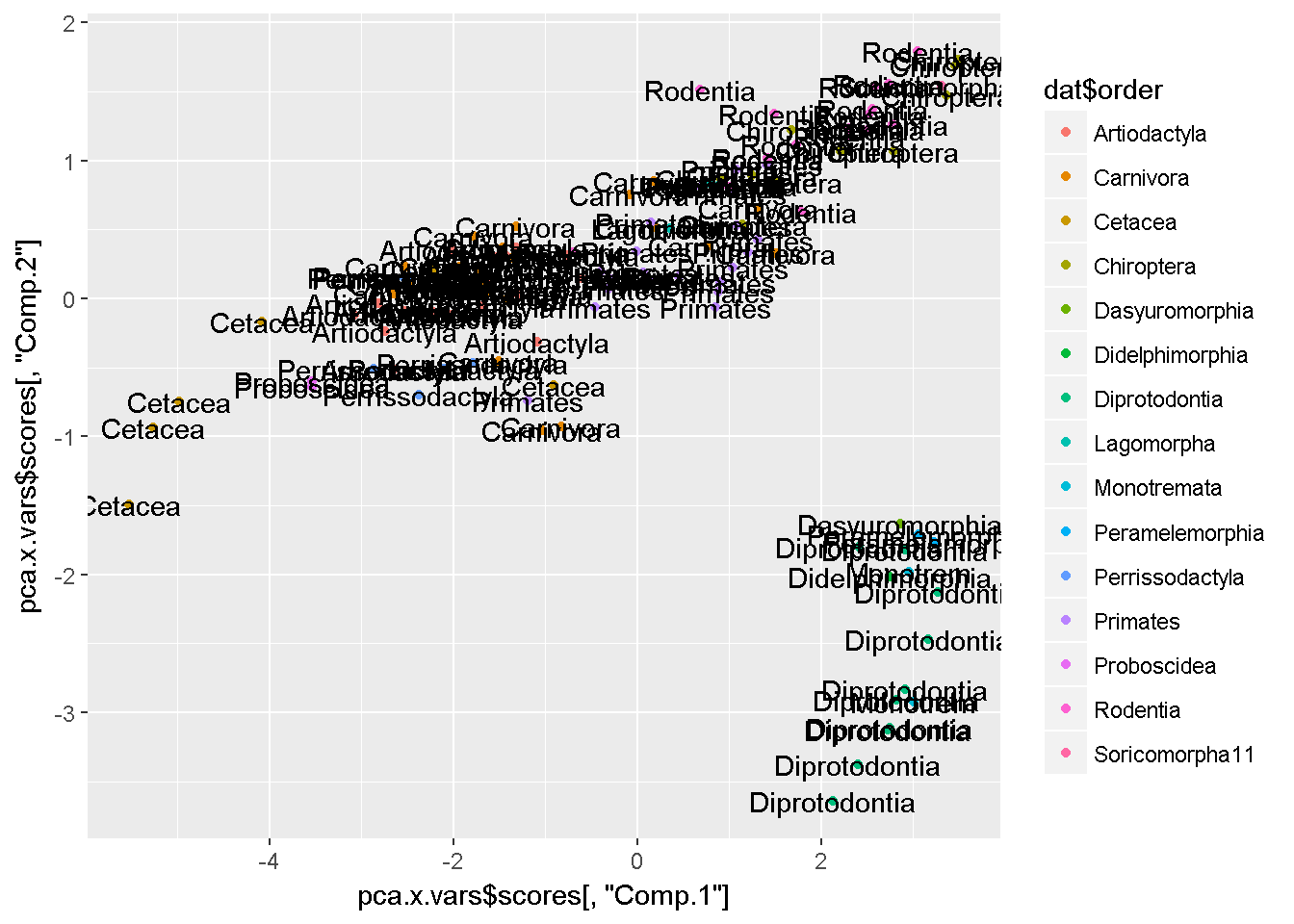Make new variable for outliers

i.weirdos <- which(dat\$order %in% c("Monotremata"  #monotremes
,"Diprotodontia"  #marsupials
,"Didelphimorphia"#opossums
,"Dasyuromorphia"#tasmanian devil)
,"Peramelemorphia" #bandicoots
))

dat\$Australia <- "other"

dat\$Australia[i.weirdos] <- "Australia"

qplot(y = pca.x.vars\$scores[ ,"Comp.2"] ,
x = pca.x.vars\$scores[ ,"Comp.1"] ,
color = dat\$Australia)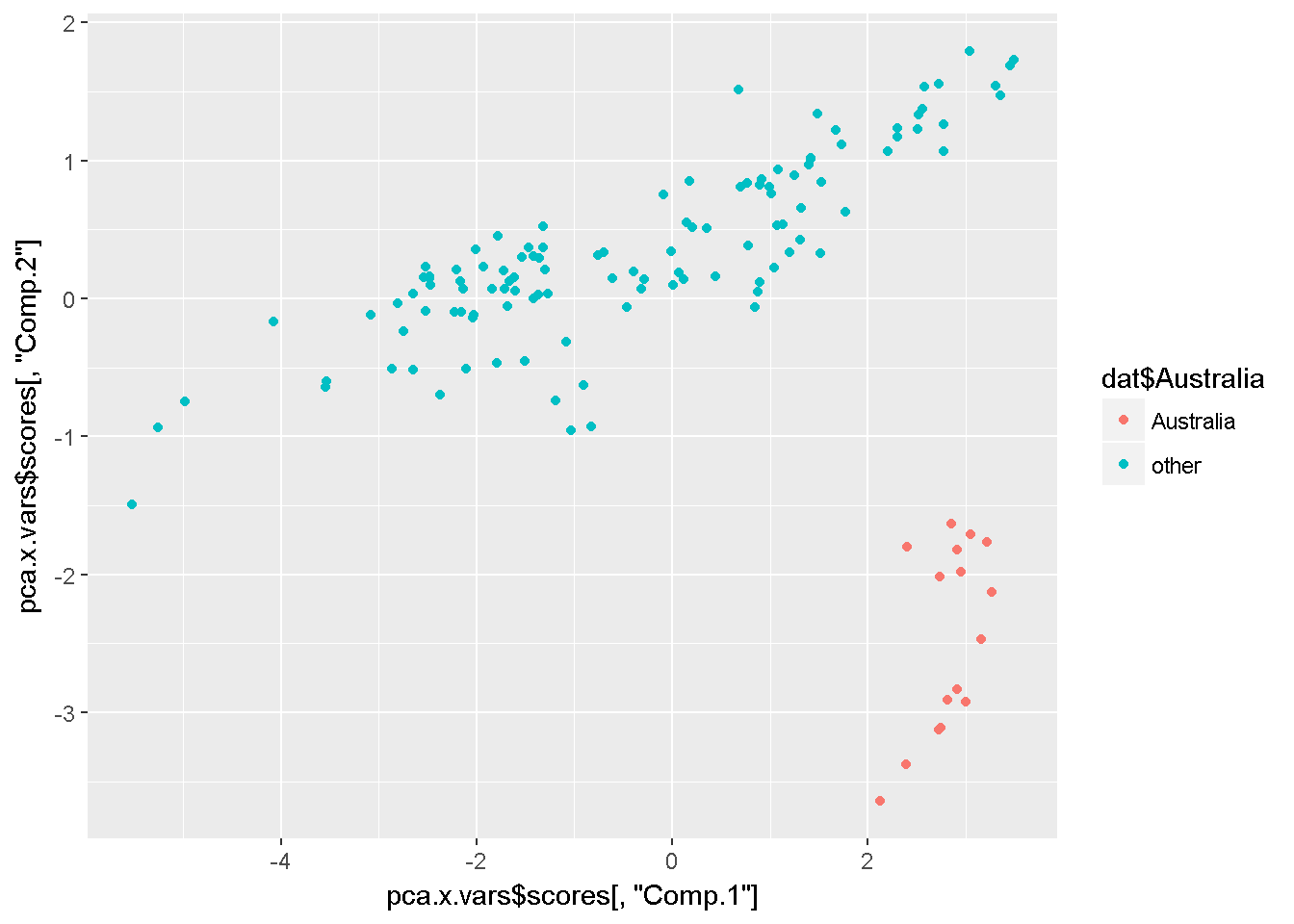As noted above, PCA generates as many latent variables as there are original variables. Usually only the first few are useful. This codes displays the 2nd vs. the 3rd PCs

qplot(y = pca.x.vars\$scores[ ,"Comp.3"] ,
x = pca.x.vars\$scores[ ,"Comp.2"] ,
color = dat\$Australia)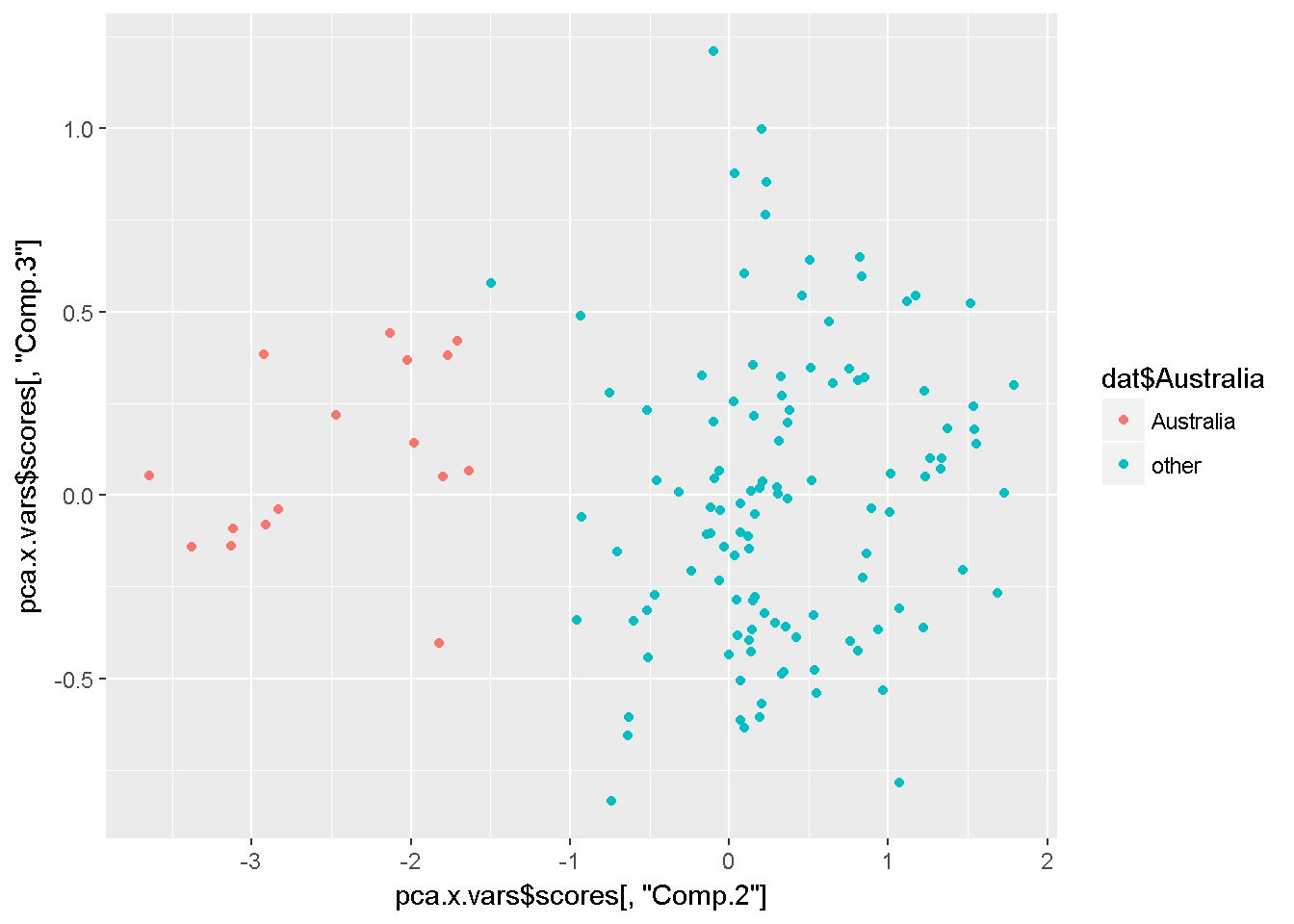And the 1st vs. the 3rd

qplot(y = pca.x.vars\$scores[ ,"Comp.3"] ,
x = pca.x.vars\$scores[ ,"Comp.1"] ,
color = dat\$Australia)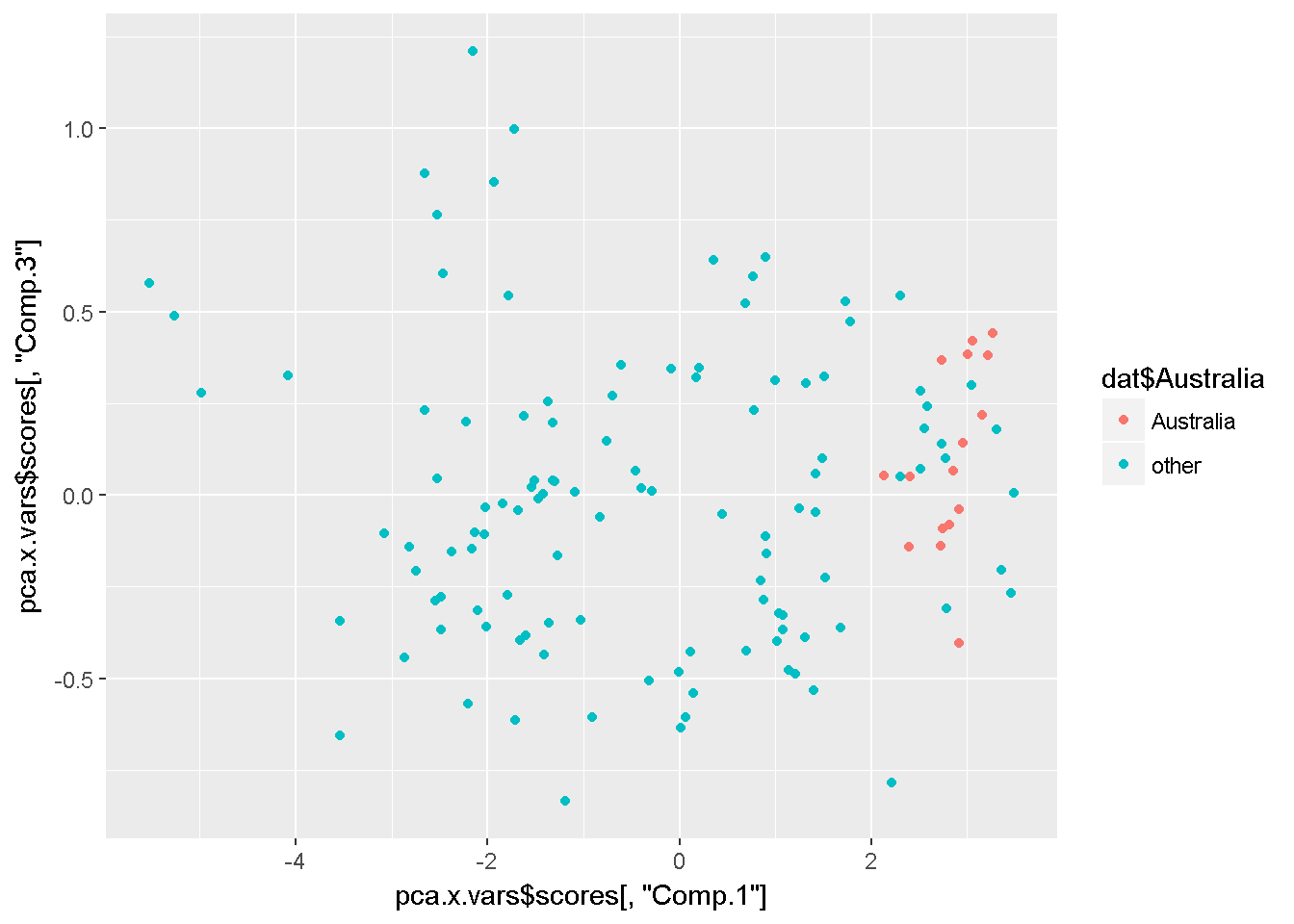PCA with response variables

This turns out to be an ugly biplot but I’ll do it anyway.

For each mammal species the authors found data on 5 different aspects of their milk

• fat %
• protein %
• sugar %
• energy
• dry.matter

These things are frequently correlated. We can see this in a “pairs” plot

pairs(dat[,c("fat","protein.num",
"sugar.num","energy.num",
"dry.matter")],lower.panel = NULL)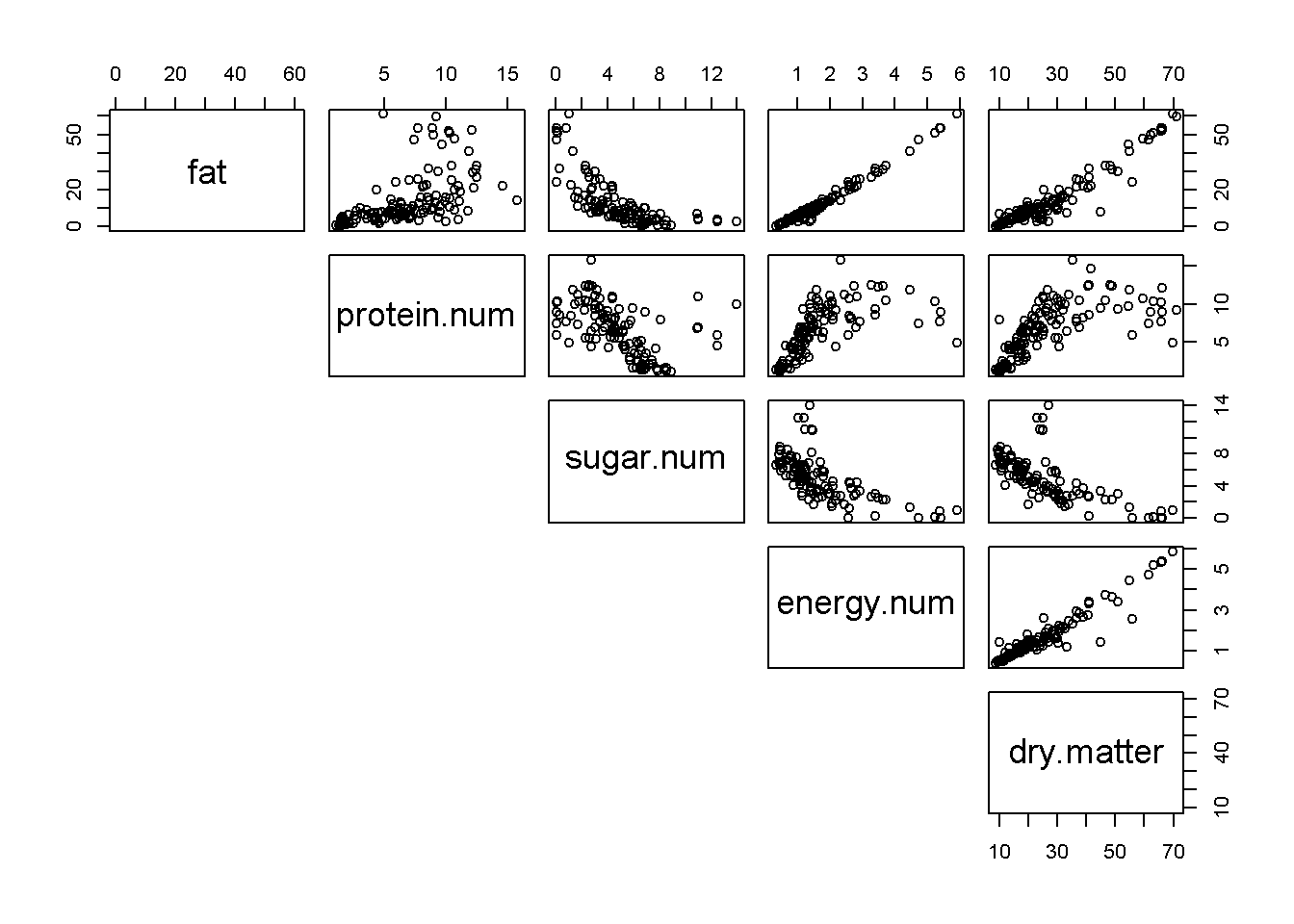You can see that the percentage data (fat, protein, sugar) are negatively correlated b/c if a high fat percent must have a low fat percent, etc. These different columns of data therefore do not have independent information. PCA allows us to combine them to look for overal axes of variation.

Run a PCA

• There are 2 base R functions for PCA
• princomp
• prcomp
• There are important differences; I forget what they are
pca.y.vars <- princomp(~ fat + protein.num +
sugar.num + energy.num +
dry.matter,
data = dat)

Make biplot of response variables

• biplots allow you to visualize axes of variation
• These response variables is not a particularly great dataset for this because the %s are all so highly correlated.
biplot(pca.y.vars, cex = 0.9,
main = "Milk y vars")